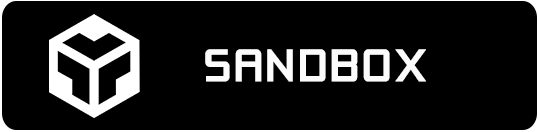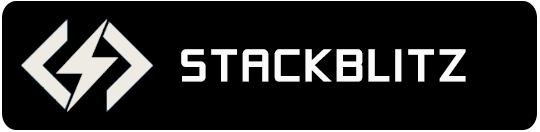# Angular Linear Gauge Overview

The Ignite UI for Angular linear gauge component allows for visualizing data in the form of a linear gauge. The `IgxLinearGaugeComponent` provides a simple and concise view of a value compared against a scale and one or more ranges. It supports one scale, one set of tick marks and one set of labels. The component has also a built-in support for animated transitions. This animation is easily customizable by setting the `transitionDuration` property. The features of the linear gauge component include configurable orientation and direction, configurable visual elements such as the needle, and more.

## Angular Linear Gauge Example

The following sample demonstrates how setting multiple properties on the same `IgxLinearGaugeComponent` can transform it to completely different linear gauge.## Dependencies

When installing the Angular gauge component, the core package must also be installed.

```npm install --save igniteui-angular-core
npm install --save igniteui-angular-gauges
```

## Component Modules

The `IgxLinearGaugeComponent` requires the following modules:

``````// app.module.ts
import { IgxLinearGaugeModule } from 'igniteui-angular-gauges';

@NgModule({
imports: [
// ...
IgxLinearGaugeModule
// ...
]
})
export class AppModule {}
``````

## Usage

The following code demonstrates how create a linear gauge containing a needle and three comparative ranges on the scale.

`````` <igx-linear-gauge width="700px"
height="30px"
minimumValue = "5"
maximumValue = "55"
value = "43">
<igx-linear-graph-range startValue="0"
endValue="15"
brush="red">
</igx-linear-graph-range>
<igx-linear-graph-range startValue="15"
endValue="30"
brush="yellow">
</igx-linear-graph-range>
<igx-linear-graph-range startValue="30"
endValue="55"
brush="green">
</igx-linear-graph-range>
</igx-linear-gauge>
``````

## Needle

This is the primary measure displayed by the linear gauge component and is visualized as a bar or you can customize it to show almost any shape as is demonstrated below.

`````` <igx-linear-gauge
height="80px" width="400px"
minimumValue=0
maximumValue=100 interval=10
value=50
isNeedleDraggingEnabled=true
needleShape="Custom"
needleBrush="DodgerBlue"
needleOutline="DodgerBlue"
needleStrokeThickness=1
needleInnerExtent=0.35
needleOuterExtent=0.65
needleOuterPointExtent=0.8
needleInnerPointExtent=0.325
needleInnerPointWidth=0
needleOuterPointWidth=0.3
needleInnerBaseWidth=0
needleOuterBaseWidth=0.07>
</igx-linear-gauge>
``````## Ranges

The ranges are visual elements that highlight a specified range of values on a scale. Their purpose is to visually communicate the qualitative state of the performance bar measure, illustrating at the same times the degree to which it resides within that state.

``````<igx-linear-gauge
height="80px" width="400px"
minimumValue=0 value=50
maximumValue=100 interval=10
rangeBrushes="#a4bd29, #F86232"
rangeOutlines="#a4bd29, #F86232" >
<igx-linear-graph-range
startValue=0 endValue=50
innerStartExtent=0.075 innerEndExtent=0.075
outerStartExtent=0.25 outerEndExtent=0.4>
</igx-linear-graph-range>
<igx-linear-graph-range
startValue=50 endValue=100
innerStartExtent=0.075 innerEndExtent=0.075
outerStartExtent=0.4 outerEndExtent=0.55>
</igx-linear-graph-range>
</igx-linear-gauge>
``````## Tick Marks

The tick marks serve as a visual division of the scale into intervals in order to increase the readability of the linear gauge.

Major tick marks – The major tick marks are used as primary delimiters on the scale. The frequency they appear at, their extents and style can be controlled by setting their corresponding properties.

Minor tick marks – The minor tick marks represent helper tick marks, which might be used to additionally improve the readability of the scale and can be customized in a way similar to the major ones.

``````<igx-linear-gauge
height="80px" width="400px"
minimumValue=0 value=50
maximumValue=100
interval=10
tickBrush="DodgerBlue"
ticksPreTerminal=0
ticksPostInitial=0
tickStrokeThickness=2
tickStartExtent=0.25
tickEndExtent=0.05
minorTickCount=4
minorTickBrush="DarkViolet"
minorTickEndExtent=0.05
minorTickStartExtent=0.15
minorTickStrokeThickness=1>
</igx-linear-gauge>
``````## Labels

The labels indicate the measures on the scale.

``````<igx-linear-gauge
height="80px" width="400px"
minimumValue=0 value=50
maximumValue=100 interval=10
labelInterval=10
labelExtent=0.025
labelsPreTerminal=0
labelsPostInitial=0
fontBrush="DodgerBlue"
font="11px Verdana">
</igx-linear-gauge>
``````## Backing

The backing element represents background and border of the linear gauge component. It is always the first element rendered and all the rest of elements such as labels, and tick marks are overlaid on top of it.

``````<igx-linear-gauge
height="80px" width="400px"
minimumValue=0 value=50
maximumValue=100 interval=10
backingBrush="#bddcfc"
backingOutline="DodgerBlue"
backingStrokeThickness=4
backingInnerExtent=0
backingOuterExtent=1>
</igx-linear-gauge>
``````## Scale

The scale is a visual element that highlights the full range of values in the linear gauge. You can customize the appearance and the shape of the scale. It can also be inverted (using `isScaleInverted` property) and all labels will be rendered from right-to-left instead of left-to-right.

``````<igx-linear-gauge
height="80px" width="400px"
minimumValue=0 value=50
maximumValue=100 interval=10
isScaleInverted=false
scaleBrush="DodgerBlue"
scaleOutline="DarkViolet"
scaleStrokeThickness=1
scaleInnerExtent=0.05
scaleOuterExtent=0.65
scaleStartExtent=0.05
scaleEndExtent=0.95>
</igx-linear-gauge>
``````## Summary

For your convenience, all above code snippets are combined into one code block below that you can easily copy to your project and see the linear gauge with all features and visuals enabled.

``````<igx-linear-gauge
height="80px" width="400px"
minimumValue=0
maximumValue=100

labelInterval=10
labelExtent=0.025
labelsPreTerminal=0
labelsPostInitial=0
fontBrush="Black"
font="11px Verdana"

interval=10
tickBrush="Black"
ticksPreTerminal=0
ticksPostInitial=0
tickStrokeThickness=2
tickStartExtent=0.25
tickEndExtent=0.05

minorTickCount=4
minorTickBrush="Black"
minorTickEndExtent=0.05
minorTickStartExtent=0.15
minorTickStrokeThickness=1

value=50
isNeedleDraggingEnabled=true
needleShape="Custom"
needleBrush="Black"
needleOutline="Black"
needleStrokeThickness=1
needleInnerExtent=0.35
needleOuterExtent=0.65
needleOuterPointExtent=0.8
needleInnerPointExtent=0.325
needleInnerPointWidth=0
needleOuterPointWidth=0.3
needleInnerBaseWidth=0
needleOuterBaseWidth=0.07

isScaleInverted=false
scaleBrush="Gray"
scaleOutline="Gray"
scaleStrokeThickness=1
scaleInnerExtent=0.05
scaleOuterExtent=0.65
scaleStartExtent=0.05
scaleEndExtent=0.95

backingBrush="#cecece"
backingOutline="#cecece"
backingStrokeThickness=4
backingInnerExtent=0
backingOuterExtent=1

rangeBrushes ="#C62828, #F96232, #FF9800"
rangeOutlines="#C62828, #F96232, #FF9800">
<igx-linear-graph-range
startValue=0 endValue=50
innerStartExtent=0.075 innerEndExtent=0.075
outerStartExtent=0.25 outerEndExtent=0.4>
</igx-linear-graph-range>
<igx-linear-graph-range
startValue=50 endValue=100
innerStartExtent=0.075 innerEndExtent=0.075
outerStartExtent=0.4 outerEndExtent=0.55>
</igx-linear-graph-range>
</igx-linear-gauge>
``````

## API References

The following is a list of API members mentioned in the above sections: